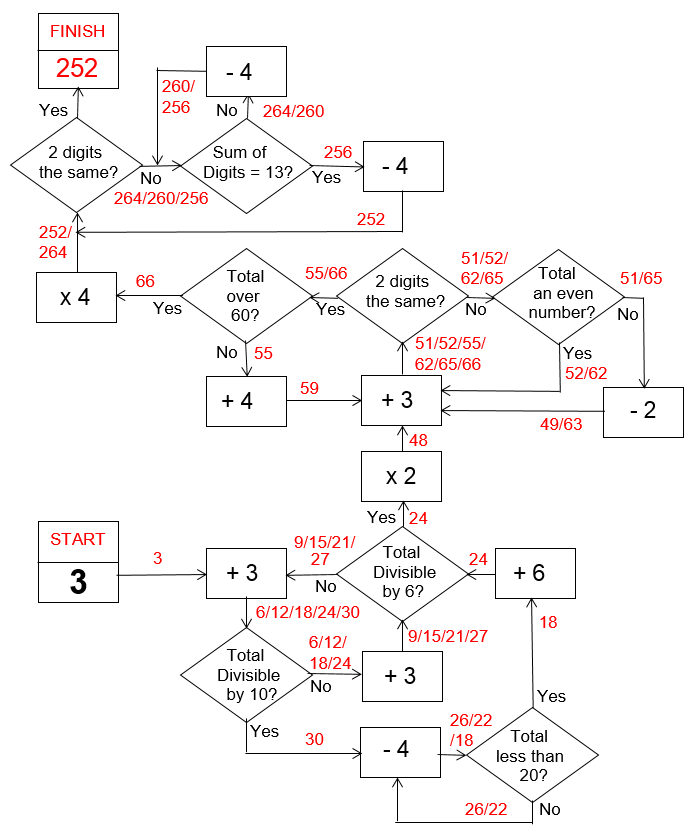Select Page

Each month, a new set of puzzles will be posted.  Come back next month for the solutions and a new set of puzzles, or subscribe to have them sent directly to you.

## MIND-Xpander Logic puzzle

There are five people in a room and each shakes every other person’s hand exactly one time. How many handshakes will there be?

## ROMAN Arithmetic Puzzles

Solve the following arithmetic problems and state your answers in Roman Numerals. Letters representing numbers in the Roman numerical system: I = 1, V = 5, X = 10, L = 50, C = 100, D = 500, M = 1,000. In this system, a letter placed after another of greater value adds (thus XVI is 16) whereas a letter placed before another of greater value subtracts (thus XC is 90). Example: 2017 = MMXVII

A) MMCCLXV – CXXII – XLIV – XCIX = ?

B) CCCXXI + DCLIV + CMLIIIVII = ?

C) DIII + XDXII + CMXXIV + CLXXI = ?

D) DCLXVI – DVI + DLV + CCCXXXIII + CDXLIV = ?

## HEXAGON-numeric Puzzle (Level 2)

Fit the numbers 1 – 6 in each hexagon and where the hexagon segments touch each other, their numbers in these 2 segments will be the same. No number can be repeated in a hexagon. The numbers in the shaded areas are the sum of the numbers in the 2 or 4 segments that they side with. The numbers in the ‘Input’ and ‘Output’ boxes are the sum of the numbers in the 3 triangular segments that they side with. Solve the puzzle and find the ‘Output’ number (?).## Feedback

There are more than one way of doing these puzzles and may well be more than one answer.  Please let me and others know what alternatives you find by commenting below.  We also welcome general comments on the subject and any feedback you'd like to give.

If you have a question that needs a response from me or you would like to contact me privately, please use the contact form.

## Get more puzzles!

If you've enjoyed doing the puzzles, consider ordering the books;

• Book One - 150+ of the best puzzles
• Book Two - 200+ with new originals and more of your favourites

Both in a handy pocket sized format.   Click here for full details.

## ARITH-maze puzzle

In the following ARITH-maze puzzle, go to ‘START’ and proceed through this numeric maze, following instructions as provided, until you get to the ‘FINISH’ box. What number did you finish with?